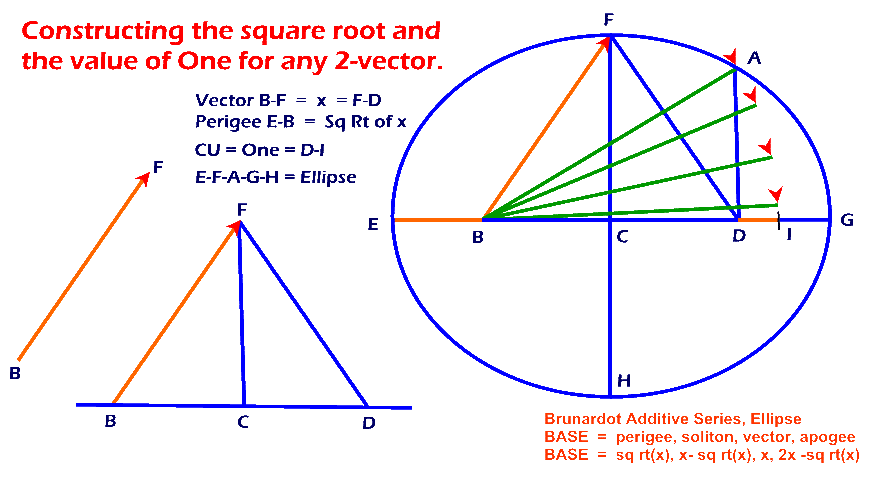Constructing the square root and the value of One for any 2-dimensional vector. The Conceptual Unit, for any system, is a constant with a value of One. Ellipses underlie all phenomena.Construct lines B-D and C-F parallel to the coordinate axes of vector B-F. Construct line F-D equal to line B-F. Construct ellipse E-F-A-G-H with focal length B-D and point F as a locus point within the ellipse. Construct line D-A at a focus parallel to axis F-H. Construct line B-A. Line B-I equals line B-A. The value of line D-I is the Conceptual Unit, One. The value of the perigee, line E-B, is the square root of vector B-F. There are several significant corollaries that apply to complex vectors, tensors, twistors, spinors, and String Theory.                                                  Brunardot